Maharashtra Board Practice Set 11 Class 7 Maths Solutions Chapter 3 HCF and LCM

Balbharti Maharashtra State Board Class 7 Maths Solutions covers the 7th Std Maths Practice Set 11 Answers Solutions Chapter 3 HCF and LCM.

HCF and LCM Class 7 Practice Set 11 Answers Solutions Chapter 3

Question 1.
Factorize the following numbers into primes:
i. 32
ii. 57
iii. 23
iv. 150
v. 216
vi. 208
vii. 765
viii. 342
ix. 377
x. 559
Solution:
i. 32∴ 32 = 2 × 2 × 2 × 2 × 2

ii. 57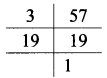∴ 57 = 3 × 19

iii. 23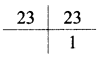∴ 23 = 23 × 1

iv. 150∴ 150 = 2 × 3 × 5 × 5

v. 216∴ 216 = 2 × 2 × 2 × 3 × 3 × 3

vi. 208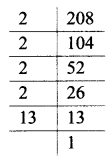∴ 208 = 2 × 2 × 2 × 2 × 13

vii. 765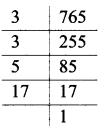∴ 765 = 3 × 3 × 5 × 17

viii. 342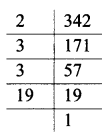∴ 342 = 2 × 3 × 3 × 19

ix. 377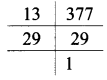∴ 377 = 13 × 29

x. 559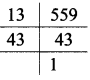∴ 559 = 13 × 43

Scroll to Top